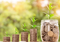## What does my light cost to run?

With lights being the most energy-consuming piece of equipment in your grow room, we're going to be addressing a significant chunk of your monthly electricity bill here.

You can either go through our simple mathematical equation, or click on this link to download our electricity cost calculator we’ve created to determine the cost of running any given light over a grow cycle and see how much you’ll save on electricity alone. You can also compare multiple lights to see a long term comparison of any of our lights.

## Electricity cost equation

Let’s assume your energy supplier is charging you 15p per kw/h. That means, if you pull exactly 1000 watts of electricity from the wall, over the space of an hour, it’ll cost you 15p.

So then, here’s how that looks as an equation if we wanted to know how much this would cost us over ten weeks, while on a 12/12 flowering cycle….

P -- Actual draw in watts (power) e.g. 670

kwh -- The price (in pounds) charged to you by your energy supplier for every hour that  you consume 1000 watts. For example, 15p kw/h is represented as 0.15

h –- The number of hours per day the unit is in use for. E.g. 12 when in flower.

d–- The number of days per week the units is in use for. This will nearly always be 7.

w–- The total weeks that the unit will be in use for, on the same timer setting. E.g. 9 when in flower.

## P×kwh×h×d×w/1000=£

The forward slash just means that we’re dividing the number we get after multiplying everything together before it, by 1000 to get the total amount in pounds

-------------------------------------------------------------------------------------------------------

What if I want to work out the price for a grow cycle that includes both veg and flower?

Simple! It’s just a case of doing two calculations; one where you include for example 18 hours ( ) and 4 weeks ( ) in one equation, and then 12 hours and 9 weeks in the other. Then you just add those two totals together.

600w HPS example

We’re now going to give you an example of what a traditional HPS light will cost you over a ten-week flowering cycle. Remember that your 600w HPS will be using more than 600 watts of electricity. See our blog ‘HPS – how much electricity do they really use?’ to find out exactly what we’re talking about. For our example below, we’re using a common wattage draw for a 600w HPS, which is around 670w.

## 670×0.15×12×7×10= 84,420(pence)

Now let’s take the number we’ve ended up with and divide it by 1,000 to get the total in pounds

84,420 ÷ 1000 = £84.42

So this means that your ‘600w’ light will cost you a total of £84.42 in electricity over a ten week cycle.

To measure the exact wattage being used in your grow we recommend checking the wattage of each unit in use with a plug-in watt meter and then include all those values into your calculations.

Again, the electricity cost calculator we’ve created makes this process much easier. You really should try it out for yourself.